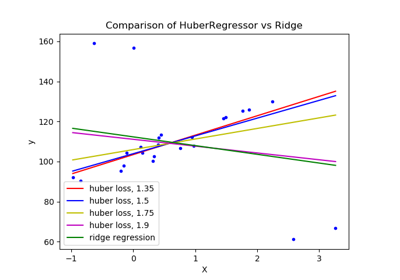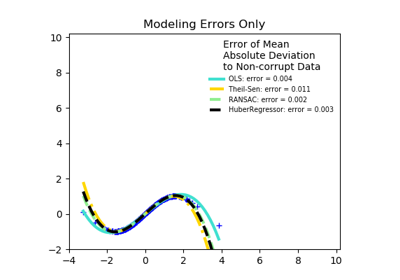# sklearn.linear_model.HuberRegressor¶

class sklearn.linear_model.HuberRegressor(epsilon=1.35, max_iter=100, alpha=0.0001, warm_start=False, fit_intercept=True, tol=1e-05)[source]

Linear regression model that is robust to outliers.

The Huber Regressor optimizes the squared loss for the samples where |(y - X'w) / sigma| < epsilon and the absolute loss for the samples where |(y - X'w) / sigma| > epsilon, where w and sigma are parameters to be optimized. The parameter sigma makes sure that if y is scaled up or down by a certain factor, one does not need to rescale epsilon to achieve the same robustness. Note that this does not take into account the fact that the different features of X may be of different scales.

This makes sure that the loss function is not heavily influenced by the outliers while not completely ignoring their effect.

Read more in the User Guide

New in version 0.18.

Parameters
epsilonfloat, greater than 1.0, default 1.35

The parameter epsilon controls the number of samples that should be classified as outliers. The smaller the epsilon, the more robust it is to outliers.

max_iterint, default 100

Maximum number of iterations that scipy.optimize.minimize(method="L-BFGS-B") should run for.

alphafloat, default 0.0001

Regularization parameter.

warm_startbool, default False

This is useful if the stored attributes of a previously used model has to be reused. If set to False, then the coefficients will be rewritten for every call to fit. See the Glossary.

fit_interceptbool, default True

Whether or not to fit the intercept. This can be set to False if the data is already centered around the origin.

tolfloat, default 1e-5

The iteration will stop when max{|proj g_i | i = 1, ..., n} <= tol where pg_i is the i-th component of the projected gradient.

Attributes
coef_array, shape (n_features,)

Features got by optimizing the Huber loss.

intercept_float

Bias.

scale_float

The value by which |y - X'w - c| is scaled down.

n_iter_int

Number of iterations that scipy.optimize.minimize(method="L-BFGS-B") has run for.

Changed in version 0.20: In SciPy <= 1.0.0 the number of lbfgs iterations may exceed max_iter. n_iter_ will now report at most max_iter.

outliers_array, shape (n_samples,)

A boolean mask which is set to True where the samples are identified as outliers.

References

Re4616ef910fb-1

Peter J. Huber, Elvezio M. Ronchetti, Robust Statistics Concomitant scale estimates, pg 172

Re4616ef910fb-2

Art B. Owen (2006), A robust hybrid of lasso and ridge regression. https://statweb.stanford.edu/~owen/reports/hhu.pdf

Examples

>>> import numpy as np
>>> from sklearn.linear_model import HuberRegressor, LinearRegression
>>> from sklearn.datasets import make_regression
>>> rng = np.random.RandomState(0)
>>> X, y, coef = make_regression(
...     n_samples=200, n_features=2, noise=4.0, coef=True, random_state=0)
>>> X[:4] = rng.uniform(10, 20, (4, 2))
>>> y[:4] = rng.uniform(10, 20, 4)
>>> huber = HuberRegressor().fit(X, y)
>>> huber.score(X, y)
-7.284608623514573
>>> huber.predict(X[:1,])
array([806.7200...])
>>> linear = LinearRegression().fit(X, y)
>>> print("True coefficients:", coef)
True coefficients: [20.4923...  34.1698...]
>>> print("Huber coefficients:", huber.coef_)
Huber coefficients: [17.7906... 31.0106...]
>>> print("Linear Regression coefficients:", linear.coef_)
Linear Regression coefficients: [-1.9221...  7.0226...]


Methods

 fit(self, X, y[, sample_weight]) Fit the model according to the given training data. get_params(self[, deep]) Get parameters for this estimator. predict(self, X) Predict using the linear model. score(self, X, y[, sample_weight]) Return the coefficient of determination R^2 of the prediction. set_params(self, \*\*params) Set the parameters of this estimator.
__init__(self, epsilon=1.35, max_iter=100, alpha=0.0001, warm_start=False, fit_intercept=True, tol=1e-05)[source]

Initialize self. See help(type(self)) for accurate signature.

fit(self, X, y, sample_weight=None)[source]

Fit the model according to the given training data.

Parameters
Xarray-like, shape (n_samples, n_features)

Training vector, where n_samples in the number of samples and n_features is the number of features.

yarray-like, shape (n_samples,)

Target vector relative to X.

sample_weightarray-like, shape (n_samples,)

Weight given to each sample.

Returns
selfobject
get_params(self, deep=True)[source]

Get parameters for this estimator.

Parameters
deepbool, default=True

If True, will return the parameters for this estimator and contained subobjects that are estimators.

Returns
paramsmapping of string to any

Parameter names mapped to their values.

predict(self, X)[source]

Predict using the linear model.

Parameters
Xarray_like or sparse matrix, shape (n_samples, n_features)

Samples.

Returns
Carray, shape (n_samples,)

Returns predicted values.

score(self, X, y, sample_weight=None)[source]

Return the coefficient of determination R^2 of the prediction.

The coefficient R^2 is defined as (1 - u/v), where u is the residual sum of squares ((y_true - y_pred) ** 2).sum() and v is the total sum of squares ((y_true - y_true.mean()) ** 2).sum(). The best possible score is 1.0 and it can be negative (because the model can be arbitrarily worse). A constant model that always predicts the expected value of y, disregarding the input features, would get a R^2 score of 0.0.

Parameters
Xarray-like of shape (n_samples, n_features)

Test samples. For some estimators this may be a precomputed kernel matrix or a list of generic objects instead, shape = (n_samples, n_samples_fitted), where n_samples_fitted is the number of samples used in the fitting for the estimator.

yarray-like of shape (n_samples,) or (n_samples, n_outputs)

True values for X.

sample_weightarray-like of shape (n_samples,), default=None

Sample weights.

Returns
scorefloat

R^2 of self.predict(X) wrt. y.

Notes

The R2 score used when calling score on a regressor will use multioutput='uniform_average' from version 0.23 to keep consistent with r2_score. This will influence the score method of all the multioutput regressors (except for MultiOutputRegressor). To specify the default value manually and avoid the warning, please either call r2_score directly or make a custom scorer with make_scorer (the built-in scorer 'r2' uses multioutput='uniform_average').

set_params(self, **params)[source]

Set the parameters of this estimator.

The method works on simple estimators as well as on nested objects (such as pipelines). The latter have parameters of the form <component>__<parameter> so that it’s possible to update each component of a nested object.

Parameters
**paramsdict

Estimator parameters.

Returns
selfobject

Estimator instance.

## Examples using sklearn.linear_model.HuberRegressor¶